1.卷积

2.转置卷积

1.卷积公式推导

2.转置卷积公式推导

3.代码演示

# 二、公式推导

## 1.卷积公式推导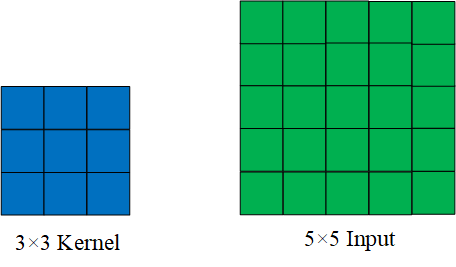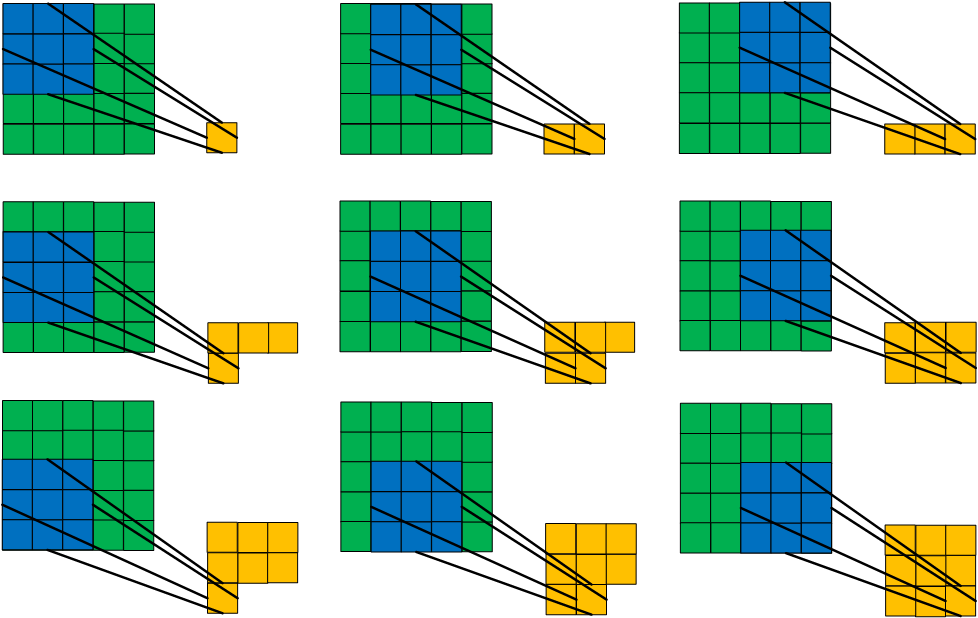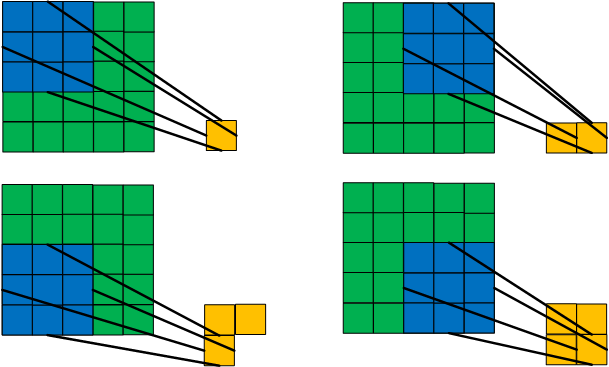（3）那么到底如何得到真正的公式呢？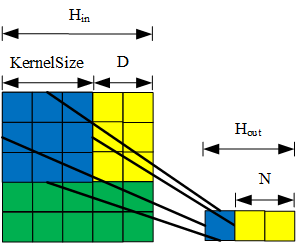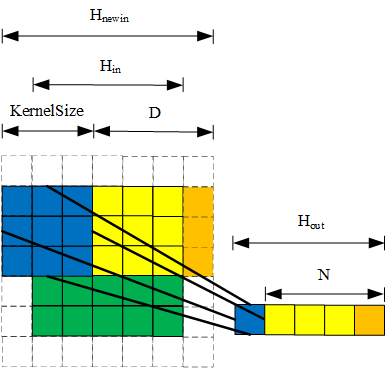## 3.代码演示

import torch
from torch.nn import Module
from torch import nn

class Net(Module):
def __init__(self):
super(Net, self).__init__()
# outsize=(2-1)*2-2*1+3=3
# outsize=(2-1)*1-2*1+3=2
# outsize=(2-1)*1-2*0+3=4
# outsize=(2-1)*2-2*0+3=5
self.trans_conv = nn.ConvTranspose2d(
in_channels=3,
out_channels=3,
kernel_size=3,
stride=2,

def forward(self, x):
x = self.trans_conv(x)
return x

model = Net()
x = torch.ones((1, 3, 2, 2))
print(model(x).size())

RuntimeError: Given input size per channel: (2 x 2). Calculated output size per channel: (0 x 0). Output size is too small...

©️2019 CSDN 皮肤主题: 技术黑板 设计师: CSDN官方博客